Name:    Online Kinetics Review

Multiple Choice
Identify the letter of the choice that best completes the statement or answers the question.

1.

Which statement concerning relative rates of reaction is correct for the decomposition of phosphine?

4 PH3(g) ® P4(g) + 6 H2(g)
 a. The rate of disappearance of PH3 is 6/4 the rate of appearance of H2. b. The rate of appearance of P4 is equal to the rate of appearance of H2. c. The rate of disappearance of PH3 is 1/4 the rate of appearance of P4. d. The rate of disappearance of PH3 is 4 times the rate of appearance of H2. e. The rate of appearance of H2 is 6 times the rate of appearance of P4.

2.

Which relationship is correct for the rate of decomposition of dinitrogen pentaoxide?

2 N2O5(g) ® 4 NO2(g) + O2(g)
 a.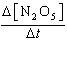=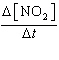=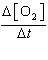b.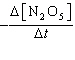==c.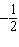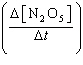=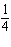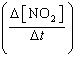d. 2=e. -2=3.

Ozone decomposes to oxygen according to the balanced chemical equation below.

2 O3(g) ® 3 O2(g)

If the rate of disappearance of ozone is -7.2 ´ 10-4 M/s, what is the rate of formation of oxygen?
 a. 4.8 ´ 10-4 M/s b. 7.2 ´ 10-4 M/s c. 1.1 ´ 10-3 M/s d. 1.4 ´ 10-3 M/s e. 2.2 ´ 10-3 M/s

4.

Which of the following changes are likely to increase the rate of a chemical reaction?

 1. Adding a catalyst 2. Increasing the temperature of the reactants 3. Increasing the concentrations of the reactants
 a. 1 only b. 2 only c. 3 only d. 1 and 3 e. 1, 2, and 3

5.

What is the name given to a substance that increases the rate of a chemical reaction but is not itself consumed?
 a. reactant b. product c. intermediate d. catalyst e. element

6.

What is the overall order of the reaction below

2 NO(g) + 2 H2(g) ® N2(g) + 2 H2O(g)

if it proceeds via the following rate expression?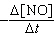= k[NO]2[H2]
 a. zero-order b. first-order c. second-order d. third-order e. fourth-order

7.

Carbon monoxide reacts with oxygen according to the equation below.

2 CO(g) + O2(g) ® 2 CO2(g)

What is the overall order of the reaction?
 a. first-order b. second-order c. third-order d. fourth-order e. not enough information is given to determine the reaction order

8.

Given the initial rate data for the decomposition reaction,

A ® B + C

determine the rate expression for the reaction.

 [A], M -D[A]/Dt M/s 0.110 7.81 ´ 10-3 0.220 3.12 ´ 10-2 0.330 7.03 ´ 10-2
 a.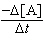= 7.10 ´ 10-2s-1[A] b.= 0.645 M-1s-1[A]2 c.= 3.63 ´ 10-2M-1s-1[A]2 d.= 3.63 ´ 10-2M s-1 e.= 5.87 M-2s-1[A]3

9.

Given the initial rate data for the reaction A + B ® C, determine the rate expression for the reaction.

 [A], M [B], M D[C]/Dt (initial) M/s 0.250 0.150 8.90 ´ 10-6 0.250 0.300 1.78 ´ 10-5 0.500 0.300 7.12 ´ 10-5
 a.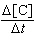= 2.37 ´ 10-4 M-1s-1 [A][B] b.= 9.49 ´ 10-4 M-2s-1 [A]2[B] c.= 1.58 ´ 10-3 M-2s-1 [A][B]2 d.= 6.33 ´ 10-3 M-3s-1 [A]2[B]2 e.= 3.80 ´ 10-3 M-3s-1 [A]3[B]

10.

For the reaction A + 2B ® C, the rate law is= k[A][B]2.

What are the units of the rate constant where time is measured in seconds?
 a.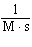b.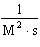c.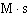d.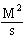e.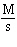11.

For the reaction 2A + B ® C, the rate law is= k[A]2[B].

Which of the factor(s) will affect the value of the rate constant for this reaction?

 1. Increasing the concentration of reactant A 2. Adding a catalyst 3. Increasing the temperature
 a. 1 only b. 2 only c. 3 only d. 2 and 3 e. 1, 2, and 3

12.

The reaction of NO and O2 produces NO2.

2 NO(g) + O2(g) ® 2 NO2(g)

The reaction is second-order with respect to NO(g) and first-order with respect to O2(g). At a given temperature, the rate constant, k, equals 6.1 ´ 103 M-2s-1. What is the rate of reaction when the initial concentrations of NO and O2 are 0.020 M and 0.015 M, respectively?
 a. 9.8 ´ 10-10 M/s b. 0.027 M/s c. 0.037 M/s d. 1.0 M/s e. 1.8 M/s

13.

How are the exponents in a rate law determined?

 1. They are equal to the concentrations of the reactants. 2. They are equal to the coefficients in the balanced chemical equation. 3. They are determined by experimentation.
 a. 1 only b. 2 only c. 3 only d. 1 and 2 e. 2 and 3

14.

For a second-order decomposition reaction,

2A ® B rate = k[A]2

which of the following functions can be plotted versus time to give a straight line?
 a. [A] b. [A]2 c. ln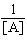d. ln[A] e.15.

A student analyzed a first-order reaction and obtained the graph below. Unfortunately, the student forgot to label the axes. What are the correct labels for the x and y axes?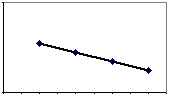a. x axis = time, y axis = ln[A] b. x axis = time, y axis = [A] c. x axis = time, y axis = 1/[A] d. x axis = 1/Time, y axis = [A] e. x axis = 1/Time, y axis = 1/[A]

16.

Which of the following equations corresponds to the integrated expression for a second-order decomposition reaction?
 a. [A] = -kt + [A]0 b.= kt +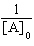c. ln[A] = -kt + ln[A]0 d.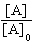= -kt e.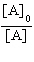= -kt

17.

The half-life of a first-order decomposition reaction is 2.36 hours. If the initial concentration of reactant is 0.52 M, what is the concentration of reactant after 752 seconds?
 a. 0.046 M b. 0.16 M c. 0.26 M d. 0.47 M e. 0.49 M

18.

For the first-order decomposition of N2O5 at 340 K, where k = 5.8 ´ 10-3 s-1, calculate the original concentration if the concentration of N2O5 is 0.41 M after 209 seconds.
 a. 0.12 M b. 0.23 M c. 0.72 M d. 1.4 M e. 1.7 M

19.

Which of the following equations corresponds to the integrated expression for a zero-order decomposition reaction?
 a. [A] = -kt + [A]0 b. ln[A] = -kt + ln[A]0 c.= kt +d.= -kt e.= -kt

20.

The reaction A ® B follows first-order kinetics with a half-life of 21.7 seconds. If the concentration of A is 0.430 M after 14.5 seconds, what is the initial concentration of A?
 a. 0271 M b. 0.287 M c. 0.644 M d. 0.683 M e. 1.59 M

21.

For the zero-order reaction below, a graph of ____ versus time will generate a straight line.

A ® B + C rate = k[A]0
 a. [A] b.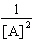c. ln[A] d.e.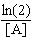22.

According to collision theory, which condition(s) must be met in order for molecules to react?

 1. The reacting molecules must collide with each other to react. 2. A catalyst must be in contact with the reacting molecules for a reaction to occur. 3. The reacting molecules must collide with an orientation that can lead to rearrangement of the atoms.
 a. 1 only b. 2 only c. 3 only d. 1 and 2 e. 1 and 3

23.

In general, as temperature increases, the rate of a chemical reaction
 a. decreases due to fewer collisions with proper molecular orientation. b. decreases for endothermic reactions. c. decreases due to an increase in the activation energy. d. increases due to a decrease in the activation energy. e. increases due to a greater number of effective collisions.

24.

The Arrhenius equation, k = A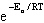relates the rate of reaction and temperature. A plot of ln(k) versus 1/T will yield a straight line with a slope of ____.
 a. -Ea/R b. -Ea c. A d.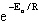e. 1/RT

25.

Molecules must overcome a barrier called the activation energy if they are to react. The highest energy point reached during the progress of a reaction is called the ____.
 a. transition state b. Arrhenius state c. half-life d. elementary step e. intermediate state

26.

For a chemical reaction, the activation energy for the forward reaction is +112 kJ and the activation energy for the backward reaction is +187 kJ. What is the overall energy change for the forward reaction?
 a. -299 kJ b. -75 kJ c. -0.599 kJ d. +75 kJ e. +299 kJ

27.

Calculate the activation energy, Ea for

N2O5(g) ® 2 NO2(g) +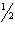O2(g)

given k (at 25.0 °C) = 3.46 ´ 10-5 s-1 and k (at 45.0 °C) = 5.79 ´ 10-4 s-1. (R = 8.314 J/K·mol)
 a. 1.32 kJ/mol b. 1.61 kJ/mol c. 14.0 kJ/mol d. 48.2 kJ/mol e. 111 kJ/mol

28.

For a given reaction, the rate constant doubles when the temperature is increased from 37 °C to 51 °C. What is the activation energy for this reaction? (R = 8.314 J/K·mol)
 a. 18 kJ/mol b. 41 kJ/mol c. 59 kJ/mol d. 86 kJ/mol e. 780 kJ/mol

29.

The effect of adding a catalyst to a reaction is to
 a. increase the number of collisions between reactants. b. increase the energy of the products. c. increase the equilibrium constant of a reaction. d. lower the activation energy of a reaction. e. decrease the enthalpy change of a reaction.

30.

The elementary steps for the catalyzed decomposition of dinitrogen monoxide are shown below.

N2O(g) + NO(g) ® N2(g) + NO2(g)
2 NO2(g) ® 2 NO(g) + O2(g)

Which of the following statements are CORRECT?

 1. The overall balanced reaction is 2 N2O(g) ® 2 N2(g) + O2(g). 2. NO(g) is a catalyst for the reaction. 3. N2(g) is a reaction intermediate.
 a. 1 only b. 2 only c. 3 only d. 1 and 2 e. 1, 2, and 3

31.

In basic solution, (CH3)3CCl reacts according to the equation below.

(CH3)3CCl + OH- ® (CH3)3COH + Cl-

The accepted mechanism for the reaction is

(CH3)3CCl ® (CH3)3C+ + Cl- (slow)
(CH3)3C+ + OH- ® (CH3)3COH (fast)

What is a rate law that is consistent with the mechanism for this reaction?
 a. rate = k[(CH3)3CCl] b. rate = k[(CH3)3CCl][OH-] c. rate = k[(CH3)3C+][OH-] d. rate = k[(CH3)3CCl][OH-]/[Cl-] e. rate = k[(CH3)3CCl] [OH-]/[Cl-]

32.

Nitrogen dioxide reacts with carbon monoxide to produce nitrogen monoxide and carbon dioxide.

NO2(g) + CO(g) ® NO(g) + CO2(g)

A proposed mechanism for this reaction is

2 NO2(g) f NO3(g) + NO(g) (fast, equilibrium)
NO3(g) + CO(g) ® NO2(g) + CO2(g) (slow)

What is a rate law that is consistent with the proposed mechanism?
 a. rate = k[NO2][CO] b. rate = k[[NO2]2[CO] c. rate = k[[NO2]2[CO]/[NO] d. rate = k[NO3][CO] e. rate = k[NO2]2

33.

For the overall reaction

A + 2B ® C

which of the following mechanisms is consistent with the rate equation below?

rate = k[A] [B]2
 a. 2A + B f I (fast)I + B ® C + A (slow) b. A + B ® I (slow)I + B ® C (fast) c. 2B ® I (slow)A + I ® C (fast) d. 2B f I (fast)I + A ® C (slow) e. A + 2B ® I (fast)I + B ® C + B (slow)